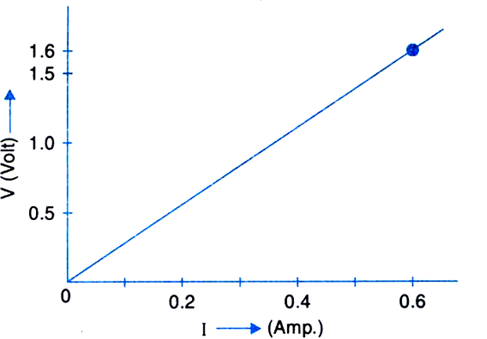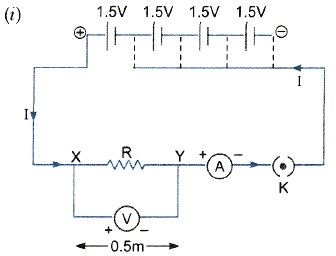(iii) What is the resistance of the wire?" ">

# An electric circuit consisting of a $0.5 \mathrm{~m}$ long nichrome wire $\mathrm{XY}$, an ammeter, a voltmeter, four cells of $1.5 \mathrm{~V}$ each and a plug key was set up.(i) Draw a diagram of this electric circuit to study the relation between the potential difference maintained between the points ${ }^{\prime} \mathrm{X}^{\prime}$ and ${ }^{\prime} \mathrm{Y}^{\prime}$ and the electric current flowing through $\mathrm{XY}$.(ii) Following graph was plotted between $V$ and $I$ values:What would be the values of $\frac{V}{I}$ ratios when the potential difference is $0.8 \mathrm{~V}, 1.2 \mathrm{~V}$ and $1.6 \mathrm{~V}$ respectively $?$ What conclusion do you draw from these values?(iii) What is the resistance of the wire?"This is the diagram of electric circuit to study the relation between the potential difference maintained between the points ${ }^{\prime} \mathrm{X}^{\prime}$ and ${ }^{\prime} \mathrm{Y}^{\prime}$ and the electric current flowing through $\mathrm{XY}$.

(ii)

When Potential, $\mathrm{V}=0.8 \mathrm{~V}$ then $, \frac{\mathrm{V}}{\mathrm{I}}=\frac{0.8}{0.3}=2.67$

At $V=1.2 \mathrm{~V},$ we have

$\frac{V}{I}=\frac{1.2}{0.45}=2.67$

At $V=1.6 \mathrm{~V},$ we have

$\frac{V}{I}=\frac{1.6}{0.6}=2.67$

As seen, ratio $\frac{V}{I}$ is constant in each case.

(iii)

The slope of the graph $\mathrm{V}$ vs. $I$ is equivalent to resistance.

Therefore, resistance of wire $X Y, R=2.67 \Omega$

Updated on: 10-Oct-2022

68 Views